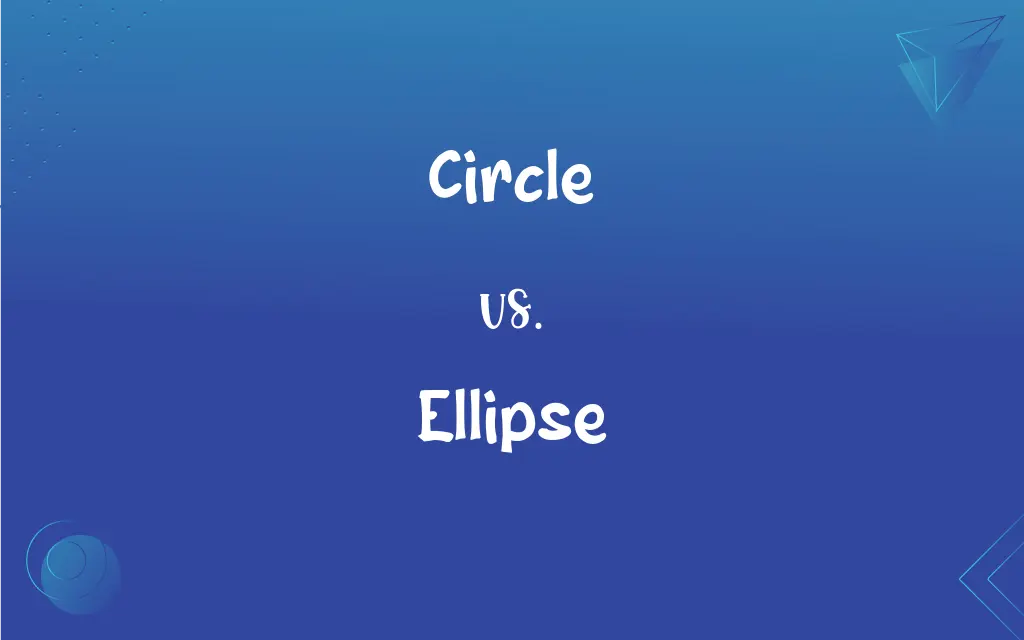# Circle vs. Ellipse: What's the Difference?Edited by Huma Saeed || By Sumera Saeed || Updated on November 18, 2023
A circle is a shape with all points equidistant from its center; an ellipse has two focal points and is elongated.## Key Differences

A circle and ellipse, both closed curves, vary distinctly in their geometric properties. A circle is a simple shape where every point on its perimeter is at an equal distance from the center. Imagine a rubber band being stretched uniformly in all directions; it forms a circle. In contrast, an ellipse appears as a "flattened" circle, having two axes of different lengths: a longer major axis and a shorter minor axis.
While every circle has a center, an ellipse boasts two focal points. For any point on an ellipse, the sum of its distances to these two foci remains constant. If these foci were to merge, the ellipse would transform into a circle. Furthermore, circles possess a consistent curvature across their entirety, providing them with perfect symmetry. Ellipses, however, exhibit varying curvature depending on the location, making them asymmetrical in comparison to circles.
While both figures are special cases of ellipses, the circle stands out as unique due to its constant radius. An ellipse, on the other hand, lacks a uniform radius, making it more complex and versatile in terms of its shape and properties.

## Comparison Chart

### Definition

All points equidistant from center
Oval shape with two focal points

### Symmetry

Perfectly symmetrical
Asymmetrical

Consistent
Varies

### Key characteristics

Two foci, major and minor axes

## Circle and Ellipse Definitions

#### Circle

A round plane figure.
The sun appears as a bright circle in the sky.

#### Ellipse

Oval shape with major and minor axes.
The racetrack was designed as an ellipse for variety.

#### Circle

Represents wholeness or completeness.
The circle of life continues endlessly.

#### Ellipse

A flattened circle with two focal points.
The orbits of planets are often in the shape of an ellipse.

#### Circle

All points equidistant from a center.
She drew a perfect circle with her compass.

#### Ellipse

A closed curve derived from intersecting a cone with a plane.
The shadow of the earth on the moon during an eclipse forms an ellipse.

#### Circle

Can symbolize infinity.
Their love was like a never-ending circle.

#### Ellipse

Sum of distances from any point to two foci is constant.
When using string and pins to draw an ellipse, the string length remains unchanged.

#### Circle

A closed curve without corners.
The kids formed a circle to play a game.

#### Ellipse

Special case of conic sections.
An ellipse becomes a circle when its foci merge.

#### Circle

A plane curve everywhere equidistant from a given fixed point, the center.

#### Ellipse

A conic section whose plane is not parallel to the axis, base, or generatrix of the intersected cone.

#### Circle

A planar region bounded by a circle.

#### Ellipse

The locus of points for which the sum of the distances from each point to two fixed points is equal.

#### Circle

Something, such as a ring, shaped like such a plane curve.

Ellipsis.

## FAQs

#### Are all round shapes circles?

No, round shapes can be circles, ellipses, or other forms.

#### What defines a circle's shape?

A circle is defined by all its points being equidistant from a central point.

#### Are all ellipses elongated?

No, when the major and minor axes are equal, an ellipse is a circle.

#### How is an ellipse formed in conic sections?

An ellipse is formed by cutting a cone with a plane at an angle to its base.

#### Why do planets have elliptical orbits?

Planetary elliptical orbits result from the gravitational forces acting between celestial bodies.

#### Which shape, circle or ellipse, is perfectly symmetrical?

A circle is perfectly symmetrical.

#### How do the major and minor axes relate to an ellipse?

An ellipse has a longer major axis and a shorter minor axis, determining its shape.

#### In what scenarios is an ellipse preferred over a circle?

Ellipses are preferred in situations requiring elongated shapes or when representing angled views of circles.

#### How does an ellipse differ from a circle in terms of foci?

A circle has one center, while an ellipse has two focal points.

#### Which shape has varying curvature: circle or ellipse?

An ellipse has varying curvature, while a circle's curvature remains consistent.

#### What's a real-world example of an ellipse?

Planetary orbits often resemble ellipses.

#### How do artists use ellipses in perspective drawing?

Artists use ellipses to depict circles viewed at an angle.

#### Can a circle be considered a type of ellipse?

Yes, a circle is a special case of an ellipse where the two foci merge into one.

#### In terms of foci, how can a circle be visualized?

A circle can be seen as an ellipse where both foci are at the same point, its center.

#### What happens when the two foci of an ellipse merge?

When the two foci merge, the ellipse becomes a circle.

#### How can one draw a perfect circle using an ellipse tool?

Using an ellipse tool, ensure the major and minor axes are equal to draw a perfect circle.

#### Which shape, circle or ellipse, can represent perfect wholeness?

A circle often symbolizes perfect wholeness or completeness.

#### Are all circles ellipses?

Yes, all circles are special cases of ellipses.

#### How do the diameters of a circle and ellipse compare?

A circle has a consistent diameter; an ellipse has varying diameters along its major and minor axes.